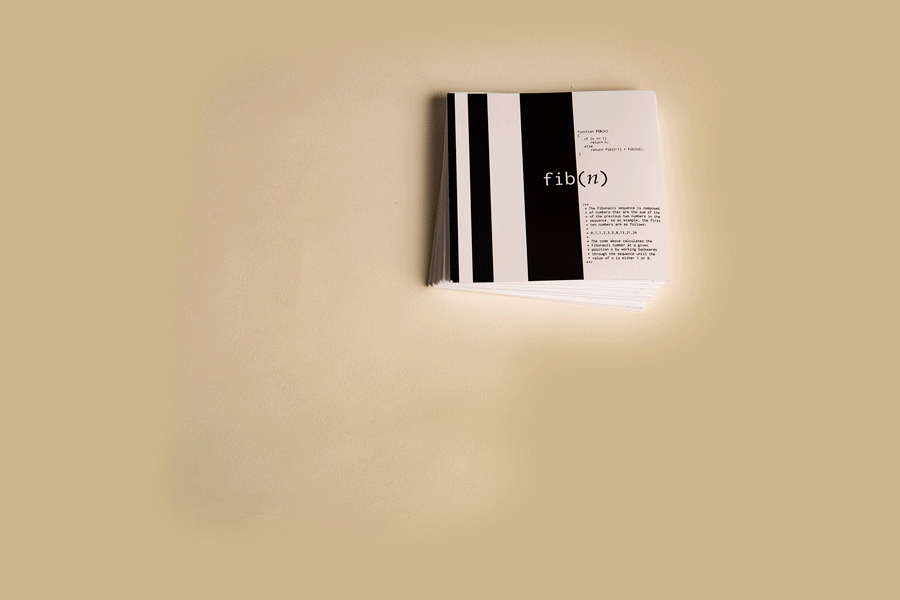# fib(n)a printed display exploring generative forms.

All forms are generated by Javascript code. The code of each form is driven by the fib(n) function, a function which produces a sequence of Fibonacci numbers.

```function fib(n){ if (n > 2) return n; else return fib(n-1) + fib(n-2); } ```

Each number in this series is the sum of the previous two. As an example, the first ten numbers are as follows:
0,1,1,2,3,5,8,13,21,34

This sequence is found in nature, often producing spiraling or branching patterns.Each Fib(n) form is accompanied by a description, the code that generates the form, and examples of the form generated under different parameters.Shown above, is a Fibonacci tree, and this is the tree at one, two and three iterations. This is a classic interpretation of the Fibonacci sequence. The forms introduced later interpret the sequence in more obscure and complex ways.